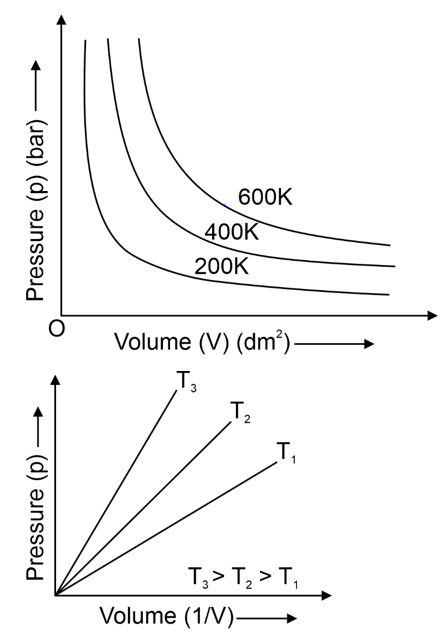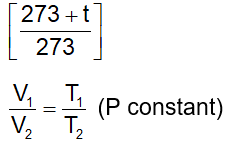# Chemistry formula for class 11 chapter- Gaseous State

## Class 11 Chapter- Gaseous State

### Units :

• St unit for volume is cubic metre (m3).
• St unit for mass is kilogram.
• St unit for pressure is Pascal (1Pa = 1N/m2).
• 1 poise = 1 g cm–1s–1 = 10–1 kg m–1 s–1
• Viscocity Units (SI) = N sm–2 or Pas.
• 1 kg is defined as the mass of a certain block of platinum-Iridium alloy preserved at Sevres out side Pairs.
• 1 atm = 76 cm of Hg = 760mm of Hg = 1.01325 × 105 N/m2
• 1 m2 Column of air extending from the earth’s surface through the upper at me sphere has a mass of about 10.300 kg. It exerts pressure 101kPa.
• T = t°C + 273 (Absolute temperature/Kelvin temperature/Thermodynamic temperature)
• S.T.P conditions

### Latest Value (at Sea Level)

Standard temperature at STP = 273.15K

Standard pressure at STP = 105Pa

Molar volume at STP = 22.71 L

### Earlier Values

Standard temperature at STP = 273.15K

Standard pressure at STP = 760 mm or 101.325 kPa.

Molar volume at STP = 22.414L

• Number of molecules in one mole og gas is 6.022 × 1023, which is also known as Avogadro’s number (NA)

### Boyle's Law

“The volume of a given mass of a gas at constant temperature is inversely proportional to the pressure” P1V1 = P2V2.

• Graphs between P & V at constant temperature are called “Isotherms”### Charles Law

“At constant pressure, the volume of a given mass of a gas at 0°C, increases or decreases by 1/273 times its volume for every degree rise or fall in temperature respectively”.

• According to Charless-Gaylussac law volume at t°C is Vt = V0### Dalton's Law

• According to Dalton's law of partial pressures, "the totalpressure exerted bya mixture ofnon- reaching gases is the sumof the individualpres- sures that each gas would exert if it were present alone in the container at the same tem- perature".
• PTotal = PA + PB+ PC + .......
• Mole fraction of gas = number of moles of one gas total number of moles in mixture
• Pressure of dry gas = observed pressure - aqueous tension.

### Kinetic Molecular Theory Of Gases

• Gases contain large number of tiny and dis- crete particles called molecules.
• The molecules move randomly in all the di-rection with high velocities.
• The volume of the molecule is negligible com- pared to the volume of the container or the mean free path is very high compared to the diameter of the molecule.
• Molecules are electrically neutral and do not have attractions or repulsions between them.
• The molecular collisions are perfectly elastic.
• Molecular motions are uneffected by gravity.
• Pressure exerted by a gas is due to the colli- sions of molecules made on the walls of the vessel. There is no loss of energy in these collisions.
• Average Kinetic Energy αT

### Liquefaction of Gases

• Intermolecular forces are inversely propor- tional to thermal energy.
• If thermal energy predominates, solid→liquid→ gas
• If intermolecular forces predominates, gas→liquid→solid
• Critical temperature (TC) is the temperature at which the gaseous state transforms continuously into the condensed state or liquid state.
• Pressure required to liquefy a gas at it's critical temperature (TC) is called critical pressure (TC)
• Volume occupied by one mole of the gas at critical temperature and critical pressure is critical volume (TC).
• Critical temp of CO2 = 31.040 C (304.19K).

### Conditions of liquefaction of gases

• A gas liquefies if it is cooled below its boiling point at given pressure. For example: Chlorine at liquefied by cooling it to -34.00C in a dry ice bath.
• For N2 and O2 that have very low boiling points -1960C and -1830C, such simple technique is not suitable. Then to liquifity such type ofgases technique based on itermolecular forces is used.
• For this the velocities of moleculesare reduced to such lower values that neighbors can at- tract each other by their interaction or inter molecular attractions, then the cooled gas will condense to a liquid.
• The molecules are allowed to expand into available volume during which the molecules overcome inter moleculear attractions by using their kinetic energies.
• The way of cooling of gas by expansion from high pressure side to low pressure is called joule-Thomson effect.

Do follow NCERT text book and solve questions with the help of Physics Wallah. NCERT solutions for Class 11 chemistry.  Students can also access the Class 11 Chemistry Notes from here and If any students need to take the online test to check their concepts or undertstanding then they can visit Quiz for States of Matter.

Download free pdf sheet which consist of formulas and important points of Gaseous State from the link given below .